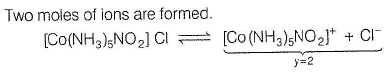Courses

# Test: Van't Hoff Factor (Liquid Solution)

## 20 Questions MCQ Test Chemistry for JEE | Test: Van't Hoff Factor (Liquid Solution)

Description
This mock test of Test: Van't Hoff Factor (Liquid Solution) for Class 12 helps you for every Class 12 entrance exam. This contains 20 Multiple Choice Questions for Class 12 Test: Van't Hoff Factor (Liquid Solution) (mcq) to study with solutions a complete question bank. The solved questions answers in this Test: Van't Hoff Factor (Liquid Solution) quiz give you a good mix of easy questions and tough questions. Class 12 students definitely take this Test: Van't Hoff Factor (Liquid Solution) exercise for a better result in the exam. You can find other Test: Van't Hoff Factor (Liquid Solution) extra questions, long questions & short questions for Class 12 on EduRev as well by searching above.
QUESTION: 1

### Only One Option Correct Type This section contains 7 multiple choice questions. Each question has four choices (a), (b), (c) and (d), out of which ONLY ONE is correct Q. The degree of dissociation (α ) o f weak electrolyte AxBy is related to van’t Hoff factor (i) by the expression [AIEEE 2011]

Solution:

(a) van’t Hoff factor (i) = [1 + (n - 1)α] where, n = number of ions from one mole of solute

α = degree of ionisation/association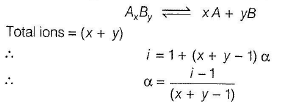QUESTION: 2

### 0.004 M Na2S04 aqueous solution is isotonic with 0.01 M glucose solution at 300 K. Thus, degree of dissociation of Na2S04 is

Solution: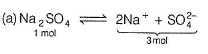i (van’t Hoff factor) = 1 + (y — 1) x = (1 + 2 x)

∴ π, (osmotic pressure) = MRTi = 0.004 x R x 300 x (1 + 2x)

Glucose is a non-electrolyte.
Hence,

i = 1

π2 = mRTi = 0.01 x R x 300 x 1 Two solutions are isotonic, hence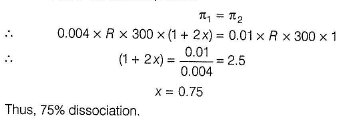QUESTION: 3

### When 20 g of naphthoic acid (C11H8O2) is dissolved in 50 g of benzene, a freezing point depression of 2 K is observed. [Kf (benzene) = 1.72Kmol-1 kg]. The van’t Hoff factor (i) is  [IIT - JEE 2007]

Solution: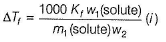w1| = 20.0 g

m1 = 11x 12 + 8 x 1 + 2 x 16 = 172 g mol-1  w2 = 50.0 g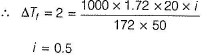QUESTION: 4

An aqueous solution of a solute AB has boiling point of 101.08° C and freezes at -1 .80 °C . AB is found to be 100% ionised at boiling point. If Kb /Kf = 0.3, then AB

Solution: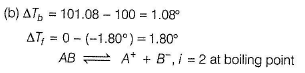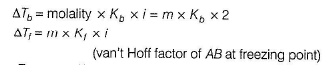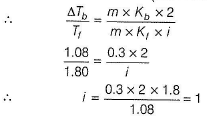Thus, AS behaves as a non-electrolyte at the freezing point of solution (remains non-ionised).

QUESTION: 5

Osmolarity of 0.02 M potassium ferrocyanide solution at 300 K is (assume solute is 100% ionised)

Solution:

Osmotic pressure is a colligative property, hence osmolarity is the molarity of the solution involving van't Hoff factor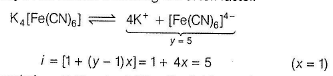Thus, osmolarity = 0.02 x i= 0.02 x 5 = 0.I0 osmol

QUESTION: 6

AB and CD are two non -volatile solutes dissolved in same solvent, showing following behaviour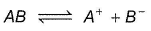AB is ionised in solvent, α = degree of ionisation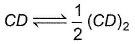CD is dimerised in solvent, 3 = degree of dimerisation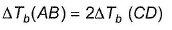If α = 0.80, then β is

Solution:

(d) For A S ,i = 1 + ( y - 1 ) a y = number of particles from one mole of solute = 2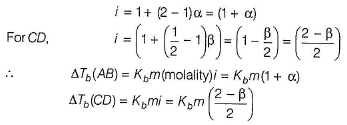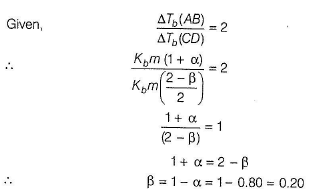QUESTION: 7

Which has the highest boiling point?

Solution:

Tb (boiling point) = T0(solvent) + ΔTb

ΔTb = m x Kb x i

m(same) = 0.1 molal

Δ Tb α i

Greater the value of i, larger the boiling point of solution.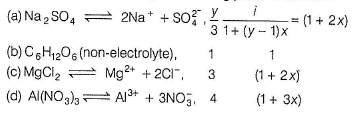*Multiple options can be correct
QUESTION: 8

One or More than One Options Correct Type
This section contains 3 multiple type questions. Each question has 4 choices (a), (b), (c) and (d), out of which ONE or MORE THAN ONE are correct.

Q.

Following solutions have been provided at temperature T K.
I. 1M aqueous glucose solution.
II. 1M aqueous sodium chloride solution.
III. 1M aqueous ammonium phosphate solution.
IV. 1M benzoic acid in benzene.
Select correct statements for the above solutions.

Solution: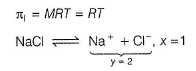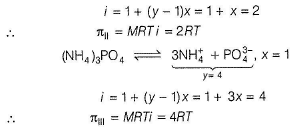In benzene, benzoic acid forms dimer by intermolecular H-bonding.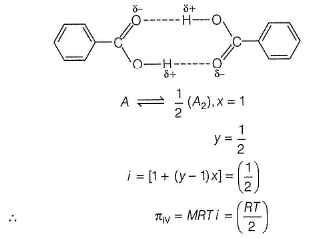Thus, osmotic pressure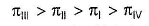Thus, solutions are not isotonic, (a) is incorrect.
III has maximum osmotic pressure, thus hypertonic of l, II and IV, (b) is correct.
IV has minimum osmotic pressure, thus hypotonic of I, II and III. Thus, (c) is correct.
II is hypotonic of III but hypertonic of I and IV, thus (d) Is correct.

*Multiple options can be correct
QUESTION: 9

Which of the following statements are correct?

Solution:

(a,c)

(a) Vapour pressure of a component in a solution,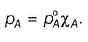by Raoult's law, thus correct.

(b) π = i MRT

For NaBr,

y = 2, / = 1 + (y - 1)x = 2

M = 1, T = (273 + t )

∴  π = 2R (273 + t) thus, incorrect.

(c)

π = i MRT

π  ∝ for equimolar solution

i = 1 for sucrose

i = (1 + x)forCH3COOH (x < < 1)

for CH3COOH being weak acid

i = 2 for KCI

i = 3 for K2S04

Thus, π (osmotic pressure) of 0.01 M solutions of sucrose < CH3COOH < KCI < K2S04 Thus, correct.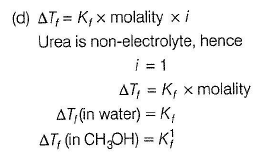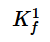and K1 are different, hence ΔTf will be different, thus incorrect.

*Multiple options can be correct
QUESTION: 10

Matching List Type
Choices for the correct combination of elements from Column I and Column II are given as options (a), (b), (c) and (d), out of which one is correct

Q.

Match the different solutions in Column I with their ΔTb in Column II and select the answer from the codes given below.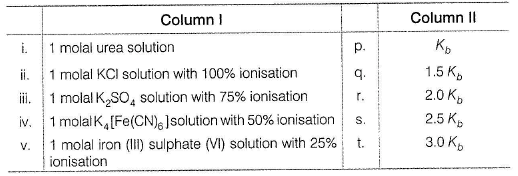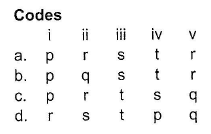Solution: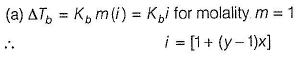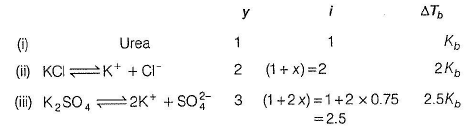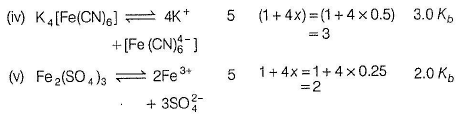Thus, (i) → (p),   (ii) → (r), (iii) → (s), (iv)→(t), (v) → (r)

*Multiple options can be correct
QUESTION: 11

In a 0.2 molal aqueous solution of a weak acid (HX), depression in freezing point is 0.383° Kf is 1.86° mol-1 kg. Assum e molarity equal to molality.
Match the parameters in Column I with their values in Column II and select the answer from the codes given below.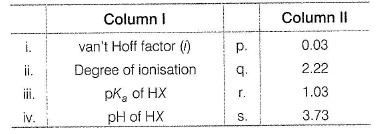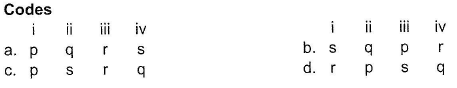Solution:

(d) Depression in freezing poin t is a colligative property.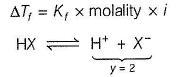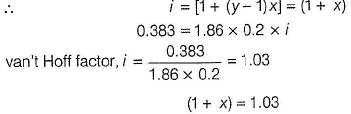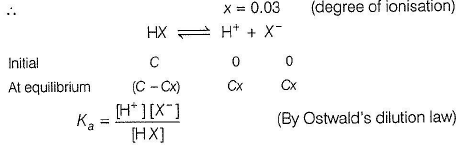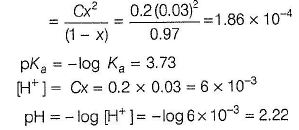Thus, (i) -> (r) (ii) -+ (p) (iii) -4 (s) (iv) -> (q)

QUESTION: 12

Comprehension Type
This section contains a passage describing theory, experiments, data, etc. Two questions related to the paragraph have been given. Each question has only one correct answer out of the given 4 options (a), (b), (c) and (d)

Passage I

Hgl2 is insoluble in water. This solute is added into Kl solution.

Q.

In the above case,

Solution: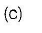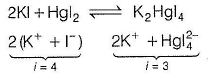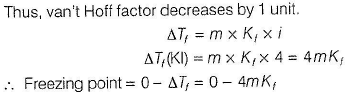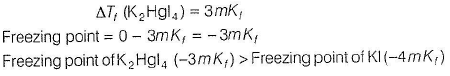QUESTION: 13

Hgl2 is insoluble in water. This solute is added into Kl solution.

Q.

Passage I van’t Hoff factor in this example

Solution:QUESTION: 14

Passage II

A solution containing 10 g of a dibasic acid in 1000 g of water freezes at -0.15° C. 10 mL of the acid is neutralised by 12 mL of 0.1 N NaOH solution. [Kf (H20 ) = 1.86° mol-1 kg]

Q.

van’t Hoff factor (/') of the dibasic acid is

Solution: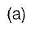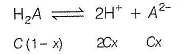y = 3 = number of ions from one unit

i = van't Hoff factor = 1 + (y - 1)x = (1 + 2x)

Also, 10 mL of dibasic acid = 12mL of 0.1 N NaOH

N1 (dibasic acid) = 0.12 g equivL-1

0.12 x equivalent weight = concentration = 10 g L-1'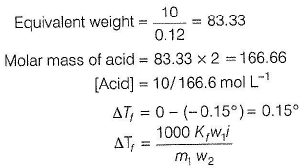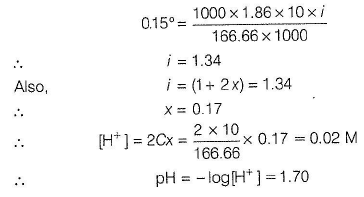QUESTION: 15

A solution containing 10 g of a dibasic acid in 1000 g of water freezes at -0.15° C. 10 mL of the acid is neutralised by 12 mL of 0.1 N NaOH solution. [Kf (H20 ) = 1.86° mol-1 kg]

Q.

pH of the solution of the acid is

Solution: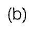y = 3 = number of ions from one unit

i = van't Hoff factor = 1 + (y - 1)x = (1 + 2x)

Also, 10 mL of dibasic acid = 12mL of 0.1 N NaOH

N1 (dibasic acid) = 0.12 g equivL-1

0.12 x equivalent weight = concentration = 10 g L-1'QUESTION: 16

Passage III

A solution containing 0.684 g of cane sugar (C12H22011) in 100 g water freezes at - 0.037° C. A solution containing 0.585 g of NaCI in 100 g water freezes at - 0.342° C.

Q.

Apparent molecular weight of NaCI is

Solution:

From ΔT, of cane sugar, K, can be determined.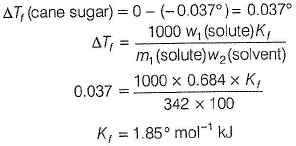For NaCI solution,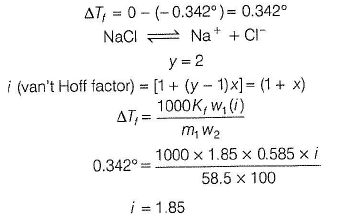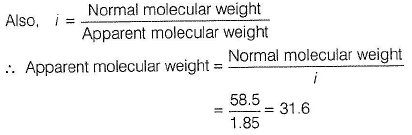QUESTION: 17

Passage III

A solution containing 0.684 g of cane sugar (C12H22011) in 100 g water freezes at - 0.037° C. A solution containing 0.585 g of NaCI in 100 g water freezes at - 0.342° C.

Q.

van’t Hoff factor (i) is

Solution:

From ΔT, of cane sugar, K, can be determined.For NaCI solution,*Answer can only contain numeric values
QUESTION: 18

Direction:

This section contains 5 questions. When worked out will result in an integer from 0 to 9 {both inclusive).

Q.

Relative decrease in vapour pressure of an aqueous NaCI solution is 0.167. Thus, number of moles of NaCI present in 180 g of H20 is ... .

Solution: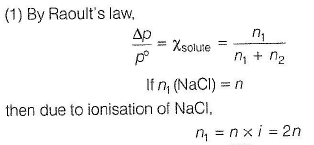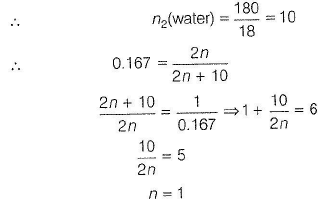*Answer can only contain numeric values
QUESTION: 19

Aqueous solution of barium phosphate which is 100% ionised has ΔTf / Kf as 0.40. Hence, given solution is x * 10-2 molal. What is the value of x?

Solution:

(8) Barium phosphate is Ba3( P04)2.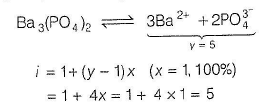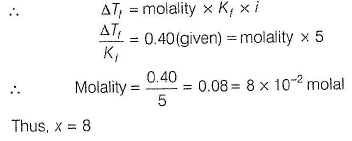*Answer can only contain numeric values
QUESTION: 20

0.002 molal aqueous solution of an ionic compound [Co(NH3)5(N02)]CI freezes at - 0.00732° C. [Kf (H20) = 1.86° mol-1kg]. How many moles of ions does 1.0 mole of the salt produce on being dissolved in water?

Solution:

ΔTf = 0 - ( - 0.00732° ) = 0.00732°
ΔTf = Molality x Kf x i

0.00732 = 0.002 x 1.86 x i

i = 1.97 = 2,0